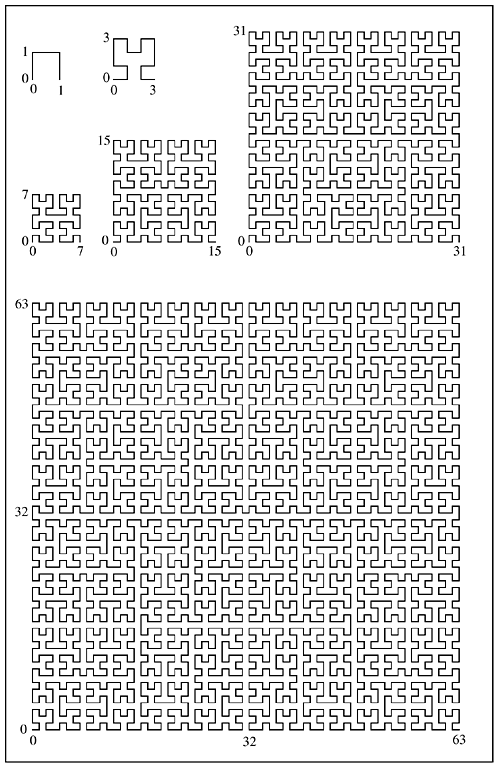14-1 A Recursive Algorithm for Generating the Hilbert Curve

To see how to generate a Hilbert curve, examine the curves in Figure 14-2. The order 1 curve goes up, right, and down. The order 2 curve follows this overall pattern. First, it makes a U-shaped curve that goes up, in net effect. Second, it takes a unit step up. Third, it takes a U-shaped curve, a step, and another U, all to the right. Finally, it takes a step down, followed by a U that goes down, in net effect.

Figure 14-2. Hilbert curves of orders 1-6.The order 1 inverted U is converted into the order 2 Y-shaped curve.

We can regard the Hilbert curve of any order as a series of U-shaped curves of various orientations, each of which, except for the last, is followed by a unit step in a certain direction. In transforming a Hilbert curve of one order to the next, each U-shaped curve is transformed into a Y-shaped curve with the same general orientation, and each unit step is transformed to a unit step in the same direction.

The transformation of the order 1 Hilbert curve (a U curve with a net direction to the right and a clockwise rotational orientation) to the order 2 Hilbert curve goes as follows:

1.       Draw a U that goes up and has a counterclockwise rotation.

2.       Draw a step up.

3.       Draw a U that goes to the right and has a clockwise rotation.

4.       Draw a step to the right.

5.       Draw a U that goes to the right and has a clockwise rotation.

6.       Draw a step down.

7.       Draw a U that goes down and has a counterclockwise rotation.

We can see by inspection that all U's that are oriented as the order 1 Hilbert curve are transformed in the same way. A similar set of rules can be made for transforming U's with other orientations. These rules are embodied in the recursive program shown in Figure 14-3 [Voor]. In this program, the orientation of a U curve is characterized by two integers that specify the net linear and the rotational directions, encoded as follows:

dir = 0: right

dir = 1: up

dir = 2: left

dir = 3: down

rot = +1: clockwise

rot = -1: counterclockwise

Figure 14-3 Hilbert curve generator.
void step(int);

void hilbert(int dir, int rot, int order) {

}

Actually, dir can take on other values, but its congruency modulo 4 is what matters.

Figure 14-4 shows a driver program and function step that is used by program hilbert. This program is given the order of a Hilbert curve to construct, and it displays a list of line segments, giving for each the direction of movement, the length along the curve to the end of the segment, and the coordinates of the end of the segment. For example, for order 2 it displays

?牋 0000牋 00 00
?牋 0001牋 01 00
?牋 0010牋 01 01
?牋 0011牋 00 01
?牋 0100牋 00 10
?牋 0101牋 00 11
?牋 0110牋 01 11
-1牋 0111牋 01 10
?牋 1000牋 10 10
?牋 1001牋 10 11
?牋 1010牋 11 11
-1牋 1011牋 11 10
-1牋 1100牋 11 01
-2牋 1101牋 10 01
-1牋 1110牋 10 00
?牋 1111牋 11 00
Figure 14-4 Driver program for Hilbert curve generator.
#include <stdio.h>
#include <stdlib.h>

int x = -1, y = 0;牋牋牋牋牋牋?// Global variables.
int i = 0;牋牋牋牋牋牋牋牋牋牋?// Dist. along curve.
int blen;牋牋牋牋牋牋牋牋牋牋牋 // Length to print.

void hilbert(int dir, int rot, int order);

void binary(unsigned k, int len, char *s) {
/* Converts the unsigned integer k to binary character
form. Result is string s of length len. */

}
void step(int dir) {

lang=EN-GB>binary(y, blen, yy);

}
int main(int argc, char *argv[]) {

}